# DCGAN with MNIST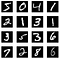Images from MNIST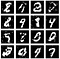Generated by a Simple GAN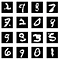Generated by DCGAN

## The Difference between the Simple GAN and the DCGAN

`generator = Sequential([        Dense(128, input_shape=(100,)),        LeakyReLU(alpha=0.01),        Dense(784),                Activation('tanh')    ], name='generator')`
`generator = Sequential([    # Layer 1    Dense(784, input_shape=(100,)),    Reshape(target_shape=(7, 7, 16)),    BatchNormalization(),    LeakyReLU(alpha=0.01),    # Layer 2    Conv2DTranspose(32, kernel_size=5, strides=2, padding='same'),     BatchNormalization(),    LeakyReLU(alpha=0.01),    # Layer 3    Conv2DTranspose(1, kernel_size=5, strides=2, padding='same'),    Activation('tanh')])`
`Layer (type)                 Output Shape              Param #   =================================================================dense_7 (Dense)              (None, 784)               79184     _________________________________________________________________reshape_5 (Reshape)          (None, 7, 7, 16)          0         _________________________________________________________________batch_normalization_7 (Batch (None, 7, 7, 16)          64        _________________________________________________________________leaky_re_lu_5 (LeakyReLU)    (None, 7, 7, 16)          0         _________________________________________________________________conv2d_transpose_4 (Conv2DTr (None, 14, 14, 32)        12832     _________________________________________________________________batch_normalization_8 (Batch (None, 14, 14, 32)        128       _________________________________________________________________leaky_re_lu_6 (LeakyReLU)    (None, 14, 14, 32)        0         _________________________________________________________________conv2d_transpose_5 (Conv2DTr (None, 28, 28, 1)         801       _________________________________________________________________activation_3 (Activation)    (None, 28, 28, 1)         0         =================================================================Total params: 93,009Trainable params: 92,913Non-trainable params: 96_________________________________________________________________`

# DCGAN with SVHN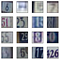Images from SVHN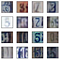Generated by DCGAN
`generator = Sequential([    # Layer 1    Dense(4*4*512, input_shape=(100,)),    Reshape(target_shape=(4, 4, 512)),    BatchNormalization(),    LeakyReLU(alpha=0.2),        # Layer 2    Conv2DTranspose(256, kernel_size=5, strides=2, padding='same'),    BatchNormalization(),    LeakyReLU(alpha=0.2),        # Layer 3    Conv2DTranspose(128, kernel_size=5, strides=2, padding='same'),    BatchNormalization(),    LeakyReLU(alpha=0.2),        # Layer 4    Conv2DTranspose(3, kernel_size=5, strides=2, padding='same'),    Activation('tanh')])`

# DCGAN with CelebA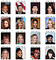Images from CelebA (Full Size)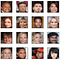Images from CelebA (Resized: 32x32)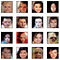Generated by DCGAN
`from keras.initializers import RandomNormalgenerator = Sequential([    # Layer 1    Dense(4*4*512, input_shape=(100,),           kernel_initializer=RandomNormal(stddev=0.02)),    Reshape(target_shape=(4, 4, 512)),    BatchNormalization(),    LeakyReLU(alpha=0.2),        # Layer 2    Conv2DTranspose(256, kernel_size=5, strides=2, padding='same',                     kernel_initializer=RandomNormal(stddev=0.02)),     BatchNormalization(),    LeakyReLU(alpha=0.2),        # Layer 3    Conv2DTranspose(128, kernel_size=5, strides=2, padding='same',                     kernel_initializer=RandomNormal(stddev=0.02)),    BatchNormalization(),    LeakyReLU(alpha=0.2),        # Layer 4    Conv2DTranspose(3, kernel_size=5, strides=2, padding='same',                     kernel_initializer=RandomNormal(stddev=0.02)),    Activation('tanh')])`

# References

## More from Naoki

https://twitter.com/naokishibuya